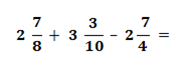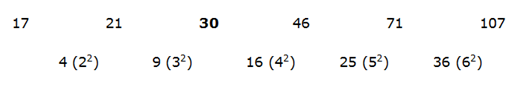# SBI Clerk Prelims 2021 Quantitative Aptitude Questions (Day-32)

Dear Aspirants, Our IBPS Guide team is providing new series of Quantitative Aptitude Questions for SBI Clerk Prelims 2020 so the aspirants can practice it on a daily basis. These questions are framed by our skilled experts after understanding your needs thoroughly. Aspirants can practice these new series questions daily to familiarize with the exact exam pattern and make your preparation effective.

Start Quiz

MISSING NUMBER SERIES

Directions (01-05): What value should come in the place of (?) in the following number series?

1) 17, 21, ?, 46, 71, 107

A.15

B.30

C.25

D.20

E.35

2) 3, 15, 35, 63, ?

A.79

B.89

C.99

D.68

E.72

3) 5, 8, 14, 26, 50, ?

A.79

B.89

C.98

D.68

E.72

4) 12, 7, ?, 13, 27

A.8

B.10

C.12

D.11

E.5

5) 3, 17, 45, ?, 143, 213

A.50

B.57

C.69

D.87

E.92

SIMPLIFICATION

Directions (06-10): What value should come in the place of (?) in the following questions?

6)A.93/40

B.97/40

C.87/40

D.69/40

E.None of these

7) 1/5 of 2675 + 24% of 1290 =?2 + 3.6

A.20

B.25

C.29

D.35

E.None of these

8) 4572 ÷ 12 * 14 – 222 =?

A.4590

B.5112

C.2567

D.3908

E.None of these

9) 4392 ÷ 24 =? – 89 * 12 + 24

A.1227

B.1567

C.2178

D.2345

E.None of these

10) 16% of 950 – 21161/2 + 20 * 14 =?

A.250

B.289

C.350

D.386

E.None of these22 – 1 = 3

42 – 1 = 15

62 – 1 = 35

82 – 1 = 63

102 – 1 = 99

5 * 2 – 2 = 8

8 * 2 – 2 = 14

14 * 2 – 2 = 26

26 * 2 – 2 = 50

50 * 2 – 2 = 98

12 * 0.5 + 1 = 7

7 * 1 + 1 = 8

8 * 1.5 + 1 = 13

13 * 2 + 1 = 272 + 3 – 2 + (7/8 + 3/10 – 7/4) =?

3 + (35 + 12 – 70)/40 =?

3 + (- 23/40) =?

3 – 23/40 =?

97/40 =?

1/5 of 2675 + 24% of 1290 =?2 + 3.6

=> 2675/5 + 24/100 * 1290 – 3.6 =?2

=> 29

4572 ÷ 12 * 14 – 222 =?

=> 381 * 14 – 222 =?

=> 5112

4392 ÷ 24 =? – 89 * 12 + 24

=> 183 =? – 1068 + 24

=> 1227

16% of 950 – 21161/2 + 20 * 14 =?

=> 152 – 46 + 280 =?

=> 386

 Check Here to View SBI Clerk Prelims 2021 Quantitative Aptitude Questions Day – 31 Day – 30 Day – 29